# Difference between revisions of "2059: Modified Bayes' Theorem"

 Modified Bayes' Theorem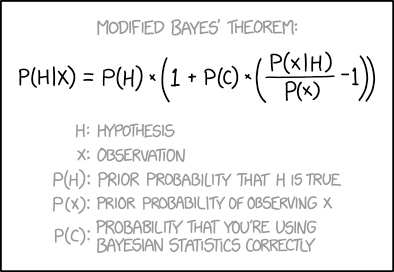Title text: Don't forget to add another term for "probability that the Modified Bayes' Theorem is correct."

## ExplanationThis explanation may be incomplete or incorrect: When using the Math-syntax please also care for a proper layout. Please edit the explanation below and only mention here why it isn't complete. Do NOT delete this tag too soon.If you can address this issue, please edit the page! Thanks.
Bayes' Theorem is an equation in statistics that gives the probability of a given hypothesis accounting not only for a single experiment or observation but also for your existing knowledge about the hypothesis, i.e. its prior probability. Randall's modified form of the equation also purports to account for the probability that you are indeed applying Bayes' Theorem itself correctly by including that as a term in the equation.

Bayes' theorem is: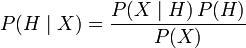$P(H \mid X) = \frac{P(X \mid H) \, P(H)}{P(X)}$, where

•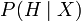$P(H \mid X)$ is the probability that$H$, the hypothesis, is true given observation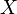$X$. This is called the posterior probability.
•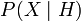$P(X \mid H)$ is the probability that observation$X$ will appear given the truth of hypothesis$H$. This term is often called the likelihood.
•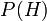$P(H)$ is the probability that hypothesis$H$ is true before any observations. This is called the prior, or belief.
•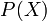$P(X)$ is the probability of the observation$X$ regardless of any hypothesis might have produced it. This term is called the marginal likelihood.

The purpose of Bayesian inference is to discover something we want to know (how likely is it that our explanation is correct given the evidence we've seen) by mathematically expressing it in terms of things we can find out: how likely are our observations, how likely is our hypothesis a priori, and how likely are we to see the observations we've seen assuming our hypothesis is true. A Bayesian learning system will iterate over available observations, each time using the likelihood of new observations to update its priors (beliefs) with the hope that, after seeing enough data points, the prior and posterior will converge to a single model.

If$P(C)=1$ the modified theorem reverts to the original Bayes' theorem (which makes sense, as a probability one would mean certainty that you are using Bayes' theorem correctly).

If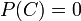$P(C)=0$ the modified theorem becomes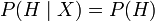$P(H \mid X) = P(H)$, which says that the belief in your hypothesis is not affected by the result of the observation (which makes sense because you're certain you're misapplying the theorem so the outcome of the calculation shouldn't affect your belief.)

This happens because the modified theorem can be rewritten as:$P(H \mid X) = (1-P(C))\,P(H) + P(C)\,\frac{P(X \mid H)\,P(H)}{P(X)}$. This is the linear-interpolated weighted average of the belief you had before the calculation and the belief you would have if you applied the theorem correctly. This goes smoothly from not believing your calculation at all (keeping the same belief as before) if$P(C)=0$ to changing your belief exactly as Bayes' theorem suggests if$P(C)=1$. (Note that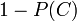$1-P(C)$ is the probability that you are using the theorem incorrectly.)

The title text suggests that an additional term should be added for the probability that the Modified Bayes Theorem is correct. But that's this equation, so it would make the formula self-referential, unless we call the result the Modified Modified Bayes Theorem (or Modified2). It could also result in an infinite regress -- we'd need another term for the probability that the version with the probability added is correct, and another term for that version, and so on. If the modifications have a limit, then we can make that the Modifiedω Bayes Theorem, but then we need another term for whether we did that correctly, leading to the Modifiedω+1 Bayes Theorem, and so on through every ordinal number. It's also unclear what the point of using an equation we're not sure of is (although sometimes we can: Newton's Laws are not as correct as Einstein's Theory of Relativity but they're a reasonable approximation in most circumstances. Alternatively, ask any student taking a difficult exam with a formula sheet.).

If we denote the probability that the Modifiedn Bayes' Theorem is correct by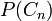$P(C_n)$, then one way to define this sequence of modified Bayes' theorems is by the rule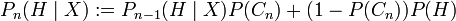$P_n(H \mid X) := P_{n-1}(H \mid X) P(C_n) + (1-P(C_n))P(H)$

One can then show by induction that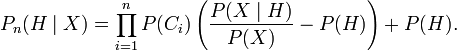$P_n(H \mid X) = \prod_{i=1}^n P(C_i)\left(\frac{P(X \mid H)}{P(X)} - P(H) \right) + P(H).$

## TranscriptThis transcript is incomplete. Please help editing it! Thanks.
Modified Bayes' theorem:
P(H|X) = P(H) × (1 + P(C) × ( P(X|H)/P(X) - 1 ))
H: Hypothesis
X: Observation
P(H): Prior probability that H is true
P(X): Prior probability of observing X
P(C): Probability that you're using Bayesian statistics correctlyadd a comment! ⋅add a topic (use sparingly)! ⋅refresh comments!

# Discussion

Right now the layout is awful:

"If$P(C)=1$ the..."
should look like this:
"If P(C)=1 the..."

But there is more wrong right now. Look at a typical Wikipedia article, the Math-extension should be used for formulas but not in the floating text. --Dgbrt (talk) 20:03, 15 October 2018 (UTC)

Credit for a good explanation though. It made perfect sense to me, even though I didn't understand it. 162.158.167.42 04:14, 16 October 2018 (UTC)
I fixed the layout and tried to enhance the explanation. --Dgbrt (talk) 20:19, 17 October 2018 (UTC)

I removed this, because it makes no sense:

As an equation, the rewritten form makes no sense.$P(H \mid X) = P(H)(1-P(C)) + P(H \mid X)P(C)$ is strangely self-referential and reduces to the piecewise equation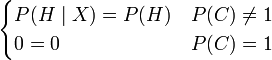$\begin{cases}P(H \mid X) = P(H) & P(C) \neq 1 \\ 0 = 0 & P(C) = 1 \end{cases}$. However, the Modified Bayes Theorem includes an extra variable not listed in the conditioning, so a person with an AI background might understand that Randal was trying to write an expression for updating$P(H \mid X)$ with knowledge of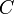$C$ i.e.$P(H \mid X,C)$, the belief in the hypothesis given the observation$X$ and the confidence that you were applying Bayes' theorem correctly$C$, for which the expression$P(H \mid X,C) = P(H)(1-P(C)) + P(H \mid X)P(C)$ makes some intuitive sense.

Between removing it and posting here, I think that I've figured out what it's saying. But it comes down to criticizing a mistake made in an earlier edit by the same editor, so I'll just fix that mistake instead.

TobyBartels (talk) 13:03, 16 October 2018 (UTC)

What about examples of correct and incorrect use of Bayes' Theorem? I don't feel equal to executing that, but DNA evidence in a criminal case could be illuminating. As a sketch, it may show that of 7 billion people alive today, the blood at the scene came from any one of just 10,000 people of which the accused is one. Interesting, but not absolute. At least 9,999 of the 10,000 are innocent. Probability of mistake or malfeasance by the testing laboratory also needs to be considered. Then there's sports drug testing, disease screening with imperfect tests and rare true positives, etc. [email protected] 141.101.76.82 09:42, 17 October 2018 (UTC)

Where is the next comic button for this page? IYN (talk) 15:46, 17 October 2018 (UTC)

Please be patient for that button, it always appears a little bit late since a few days ago when a new comic is out. Right now you always can click the Latest comic link at the menu. I'm aware of this minor issue. --Dgbrt (talk) 20:16, 17 October 2018 (UTC)